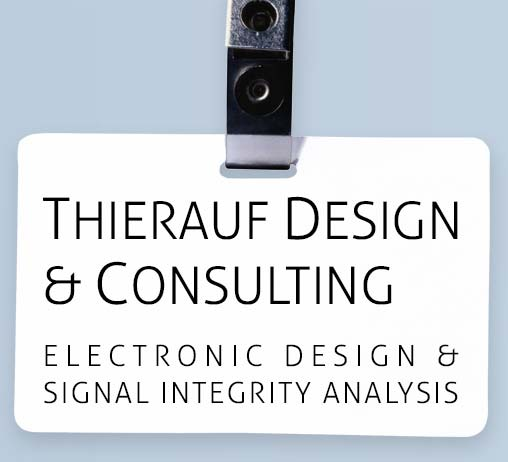#One of the most common capacitor related questions I get from clients and readers regards the way ceramic capacitors are classified.

As I've noted elsewhere, Class II ceramic dielectrics (which are used to create most ceramic decoupling capacitors, such as X7R types) are made with materials that cause the capacitance to change with temperature and bias voltage. A three character identification scheme is used to describe these effects: The 1st and 2ed characters indicate the temperature span, and the 3ed shows by how much the capacitance can change over that range. For instance, the capacitance of the popular X7R type capacitor can change by +/- 15% (indicated by the "R") when operating from -55C (indicated by the "X") to +125C (indicated by the "7"). This change is in addition to the tolerance of the capacitor, which is measured at 25C (or sometimes 20C) and low bias voltage. Operating an X7R at 50% of its working voltage rating can result in the capacitance being reduced by nearly 20%, while the capacitance can be reduced by as much as 80% for other Class II dielectrics. The identification code doesn't directly show the way voltage changes the capacitance, but in general the "X" series is the least affected, followed by the "Y" and then the "Z" series. Manufacturer's literature sometimes shows this, and it's described in detail in chapter 10 of High-Speed Circuit Board Signal Integrity. Incidentally, a Class I dielectric (such as a C0G) should be used in those situations where the capacitance must not change with bias, such as integrators, PLL's and timing circuits.

Here's what each of the most common character codes mean for Class II dielectrics (Class I dielectrics such as C0G have their own, very different, code):

X = -55C              5 = +85C              R = +/- 15%

Y = -30C               6 = +105C            U = +22%, - 56%

Z = +10C               7 = +125C           V = +22%, -82%

One more note on Class II ceramic capacitors: remember the capacitance falls as they age, even when the circuit is not powered up. The "X" series show the least amount of aging (typically under 2.5% per decade hour), while the "Z" series the most (as much as 7% in reduction of capacitance per decade hour). But that's for another post.